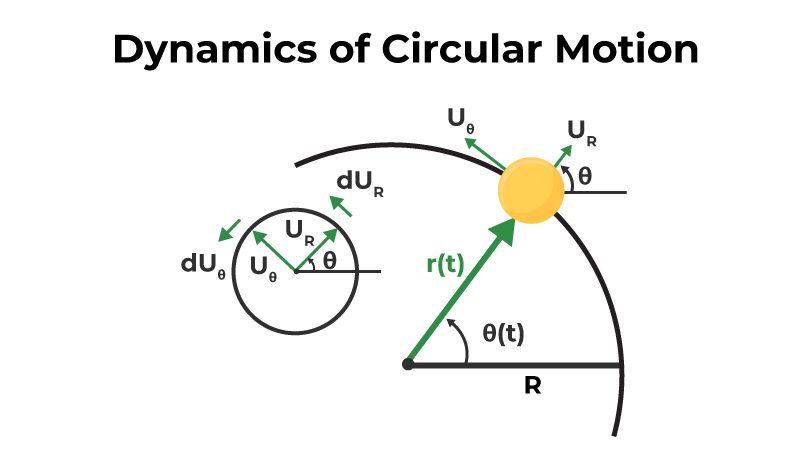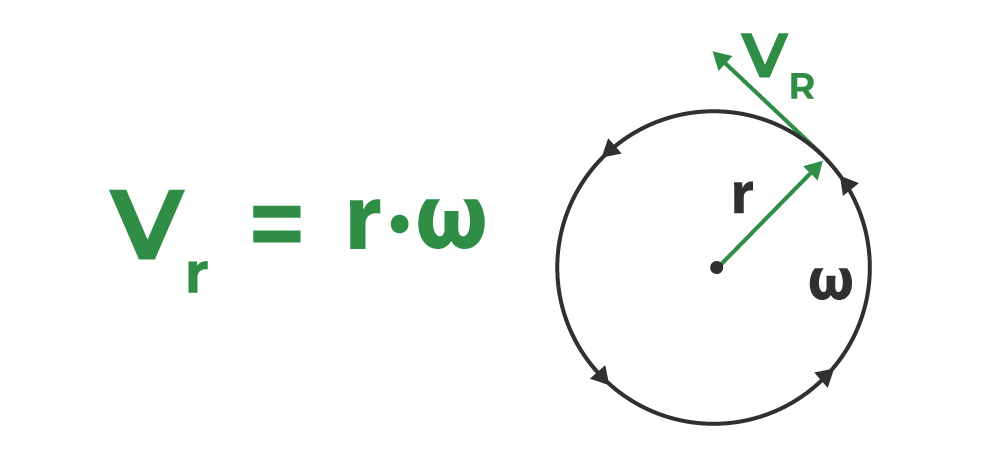GFG App
Open AppBrowser
Continue

# Dynamics of Circular Motion

Circular motion is a motion in which an object moves around a fixed point in a circular motion. It can be both uniform and non-uniform. If there is no tangential component of acceleration then it is a uniform circular motion, and if the tangential component of acceleration is present, then it is a non-uniform circular motion.

There are lots of examples around us in our daily lives where bodies perform circular motions every day. From the hands of the clock to a car turning on a banked road. All these are examples of circular motion. This motion can be classified into two categories – uniform circular motion and non-uniform circular motion. It is essential to know the dynamics and the equation of the body performing the circular motion. These dynamics allow us to analyze these motions and calculate the statistics related to the behaviour of the body in such motion.

## What is Circular Motion?

An object moving in a circular trajectory around a point is said to be performing a circular motion. For example, a car turning on the road is performing a circular motion around some centre. The circular motion of continued is periodic in nature. Periodic motions are motions that repeat themselves after a certain period of time. Based on the velocity and the acceleration, this motion can be classified into two categories –

• Uniform Circular Motion: When a body moves at constant speed in a circular motion along the circumference of the circular trajectory, it is called Uniform Circular Motion.
• Non-Uniform circular motion: When a body moves in a circular motion along the circumference of a circular trajectory in such a way that its speed keeps changing. It is called a non-uniform circular motion.

## Dynamics of Circular Motion

In this motion, the body is moving at a constant speed. Let’s say the radius of the circular trajectory on which the body is moving is “r”, and the speed of the body is v m/s. The figure shows the body going from point A to point B in time “t”. The length of the arc from point A to point B is denoted by “s”. In this, the angle covered by the object is given by,

θ = s / r

The image given below tells us about the Dynamics of Circular Motion,The angular velocity of the body is defined as the rate of change of angle. It’s similar to velocity in the case of straight-line motion.  It is denoted by the Greek symbol ω.

ω = dθ / dt

Using the relation given above for the angle covered.

ω = d / dt (s/r)

ω = ds / dt (1/r)

where,
s is the length of the arc (distance covered by the body)

v = ds / dt

where,
v is the speed of the body

Substituting the value in the equation,

ω = ds / dt (1/r)

ω = v (1/r)

v = rω

(or)

ω = v /r

The image given below shows the formula for angular velocity.## Uniform Circular Motion

Bodies have a tendency to move in a straight line. For the bodies making a circular motion at a constant speed, there must be some force that keeps them on a circular path. Such a force is called the centripetal force. The reaction of this force is called centrifugal force. This means that both these forces are equal and opposite in direction.

Centrifugal force is given by,

F = mv2 / r

We known that, ω = v / r

Substituting this relation into the equation,

F = mv2 / r

F = m(rω)2 / r

F =  mrω2

Vehicles turning on the road travel along a circular arc the forces acting on the vehicle are given below,

• Weight (Mg) of the car
• Normal reaction force (N)
• Friction

In a horizontal road, the weight of the car and normal reaction force cancel each other. The only force acting in the radial direction is friction force which provides the radial acceleration here, the force of friction acts as the centripetal force.

F = m(v2/r)

As, there is a limit to the maximum value of friction, for a safe turn,

m(v2/r) ≤ μsN

where,
µs is the coefficient of static friction

## Right-Hand Rule

While holding the axis of rotation and gripping the fingers in the direction of motion of the object. If the thumb is arranged perpendicular to the curvature of the fingers then it represents the angular displacement vector. The velocity in this motion is called angular velocity.

Angular velocity is the rate of change of angular displacement. It is denoted by ω. It is a vector quantity.

ω  = v/r

In the Right-Hand rule, the thumb represents the direction of angular velocity.

If a body performs anticlockwise rotation, then according to the right-hand rule, the direction of ω is along the axis of a rotation and directed upwards, while for clockwise rotation, ω is directed downwards.

Also, Check

## Solved Examples on Dynamics Of Circular Motion

Example 1: Find the angular velocity of a body that is moving at a speed of 10 m/s in a circle of radius 2 m.

Solution:

Formula for angular velocity is given by,

ω = v/r

Given:

v = 10m/s and r = 2 m.

ω = v/r

ω = 10/2

Example 2: Find the force acting on a particle of mass 5 kg moving in a circle of radius 4 m at an angular speed of 20 rad/s.

Solution:

Formula for centripetal force is given by,

F = mrω2

Given:

ω = 20 rad/sec, r = 2 m and m = 2Kg

F = mrω2

F = 2×2×(20)2

F = 1600 N

Example 3: An insect moves in a circle of 4 m radius and completes 20 revolutions per second. Find the angular velocity, linear velocity, and acceleration.

Solution:

The body moves at 20 revolutions per second.

ω = Angle Cover per Second
= 20(2π)
= 40π

Linear velocity is given by,

v = rω

Given:

r = 4 m

v = (4)(40π)

v= 160π m/s

Acceleration will be given by,

a = v2 / r

a = (160π)2 / 4

a = 64000 π2 m/s2

## FAQs on Dynamics Of Circular Motion

### Question 1: What is the Example of Circular Motion?

Examples of Circular motion which are encountered in our daily life are:

• Artificial Satellite revolving around the Earth.
• Motion of blades of the Windmill.
• Motion of the wheel of a Car.

### Question 2: What type of motion is called circular motion?

Circular Motion is defined as the motion of the object along the circumference of a circle i.e. the object is moving in such a way that it is always at a fixed distance from the centre of the circle.

### Question 3: What are the types of circular motion?

The two basic types of circular motion are,

• Uniform Circular Motion
• Non-Uniform Circular Motion

### Question 4: What is a centripetal force?

The force which helps an object to move on a curved path without changing its speed is called centripetal force. Some of the examples where we see centripetal acceleration are,

• When a stone is rotated by a string the force generated on the string results in centripetal acceleration.
• Gravitational force caused by the earth’s rotation around the Sun results in a centripetal force, etc.

### Question 5: What is the value of instantaneous acceleration in a uniform circular motion?

Instantaneous acceleration in a uniform circular motion is calculated as,

a = v2/R

where
v is the velocity of the object and
R is the radius of the circular path.

Direction of instantaneous acceleration is perpendicular to velocity and is directed inwards along the radius.

My Personal Notes arrow_drop_up